﻿ Regression Analysis and Seasonal Adjustment of Time Series

### Regression Analysis and Seasonal Adjustment of Time Series

Eva Ostertagová, Oskar Ostertag

Journal of Automation and Control

## Regression Analysis and Seasonal Adjustment of Time Series

Eva Ostertagová1,, Oskar Ostertag2

1Department of Mathematics and Theoretical Informatics, Faculty of Electrical Engineering and Informatics, Technical University of Košice, Nemcovej 32, 042 00 Košice, Slovak Republic

2Department of Applied Mechanics and Mechatronics, Faculty of Mechanical Engineering, Technical University of Košice, Letná 9, 042 00 Košice, Slovak Republic

### Abstract

The aim of this article is to demonstrate the dummy variables for estimation seasonal effects in a time series, to use them as inputs in a regression model for obtaining quality predictions. Model parameters were estimated using the least square method. After fitting, special tests to determine, if the model is satisfactory, were employed. The application data were analyzed using the MATLAB computer program that performs these calculations.

• Eva Ostertagová, Oskar Ostertag. Regression Analysis and Seasonal Adjustment of Time Series. Journal of Automation and Control. Vol. 3, No. 3, 2015, pp 118-121. http://pubs.sciepub.com/automation/3/3/16
• Ostertagová, Eva, and Oskar Ostertag. "Regression Analysis and Seasonal Adjustment of Time Series." Journal of Automation and Control 3.3 (2015): 118-121.
• Ostertagová, E. , & Ostertag, O. (2015). Regression Analysis and Seasonal Adjustment of Time Series. Journal of Automation and Control, 3(3), 118-121.
• Ostertagová, Eva, and Oskar Ostertag. "Regression Analysis and Seasonal Adjustment of Time Series." Journal of Automation and Control 3, no. 3 (2015): 118-121.

 Import into BibTeX Import into EndNote Import into RefMan Import into RefWorks

12
Prev Next

### 1. Introduction

If we analyze the evolution of time series, we are interested not only in the main development trend of the indicators, but also in the course and intensity of any periodic fluctuations, which these time series present. When working with time series, the data must be adjusted seasonally. The aim of seasonal adjustment is to uncover the underlying dynamics in the development of the investigated phenomena and allow a direct comparison of their development in different seasons within the year. There are many methods of seasonal adjustment and their classification is not easy, because in practice the techniques used are a combination of several methods. Often they apply different types of moving averages, which eliminate from the time series the components the frequency of which does not exceed the number of observations forming the moving average length. To eliminate seasonal component regression methods based on the theory of linear regression model are also used. In case, where the nature of the seasonal component may change, e.g. the Winters exponential smoothing is applied.

### 2. Regression Approaches to the Seasonal Component of Time Series

In the construction of the forecasts of seasonal time series, a regression model with artificial (dummy) variables with simultaneously estimated trend and seasonality parameters can be used. Artificial variable is used to quantify the effect of the respective period on the estimated value of the investigated variables. The trend component is modeled via suitable regression function, for example line, parabola, and so on. The seasonal component is expressed using artificial (zero unit) variables that assign a value to the time series unit in case it is found in the considered season and zero otherwise.

Let us assume an additive time series model in which the value of the indicator yt in the tperiod is given by the sum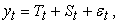where Tt is the trend component, St is the seasonal component and εt is a random component. In the presence of free parameter (constant) in the model trend, in order to avoid multicollinearity, seasonality is modeled as a qualitative variable using the s – 1 of artificial variables, where s is the length of the season included in the time series. Furthermore, we assume that the time series has a linear trend and quarterly seasonality.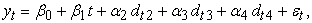(1)

where the artificial variables are defined as vectors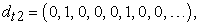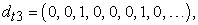(2)

of length of which is equal to n number of the time series of observations.

Since the artificial variable attains the value of one in a particular observation, we declare that in this period, to the value generated from a linear trend we shall add the value of seasonal fluctuations, which is calculated compared to the base period, which is in this case the first quarter of the year.

The artificial variableis a zero vector and the effect of the first quarter is included in the intercept β0 of the linear trend, which is interpreted in terms of the base level of the studied variables.

Model (1) contains a trend, seasonal and random component. Model parameters can be estimated using the least square method. The estimated model will take the form of:(3)

The verification of the suitability of the regression model (1) is analogous to that in any other regression model. Particularly important is to test the heteroscedasticity and autocorrelation of the random component.

The estimated regression model (3) can be used for the construction of point and interval forecasts. Forecasting requires us to choose the time variables in the horizon of h > 0 and for the seasonal variables, substitute the unit values of the respective seasons in the horizon h.

In case of the regression model with artificial variables we shall adjust the estimated trend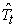and the seasonal factors,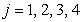, to the form of :(4)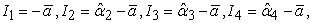(5)

where(6)

is the “average” of seasonal regression parameters.

In an analogous manner we shall proceed in case of twelve month seasonality.

Another regression method for eliminating seasonal component is based on the fact that this component is estimated by means of a suitably selected mathematical function. The most commonly used are trigonometric functions with the period length equal to the number of periods s in the year, or a fraction of this number.

Provided that the trend of the considered time series is linear, the model may have e.g. this shape for: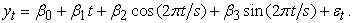(7)

Since it is a general linear regression model, estimates of the parameters may be obtained by the least square method.

In case the coefficient of determinationR2for the stated model is too small, we can continue to add to the model further unit values in the form of the considered trigonometric functions, with a half, fourth, or even smaller period, e.g.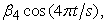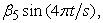etc. From the models listed we select the one for which we achieved, for example, the maximum value of the coefficient of determination and also which best meets the other criteria imposed on the linear regression model.

### 3. The Application of Regression Models with artificial Variables and trigonometric Functions at Selected Time Series

We have data available on the number of sold pieces of selected articles of a business company engaged in the Internet sales of automotive accessories for individual quarters of the year, during the period of 2008 − 2014.

Figure 1 displays the time series presented in a form of plot via line chart.

The presented graph makes clear, that the stated time series has in the respective period an increasing, approximately linear trend and quarterly seasonality. The proposed regression model with artificial variables will have the form of (1).Download asVeiw figureFigures index
Figure 1. The development of the number of articles sold in the period of 2008-2014

The model estimated by the least squares method is: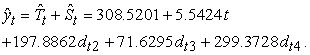(8)

For two-sided 95% confidence intervals for regression coefficients applies, that: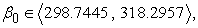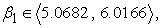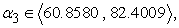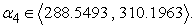To test the statistical significance of individual coefficients of the regression model the ttests were used, where we received the following result values of test statistics and pvalues:

[65.2874, 24.1790, 38.1151, 13.7565, 57.2181],

[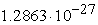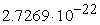,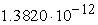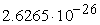].

Since p-values are in all cases below the significance level  = 0.05, all regression coefficients are considered statistically significant. The same result has also been provided by the confidence intervals for regression coefficients, since none of them contains zero value.

Based on the resulting value of the coefficient of determination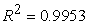we can conclude that the model explained the variability of the number of units sold of selected articles to 99.53%.

The least squares method provides unbiased point estimates of parameters of the linear regression model while meeting certain assumptions about the probability distribution of random errors εt, for, within the model.

We assume, that the random errorsεt [2, 3]:

•  have normal distribution,

•  have zero mean values, i.e.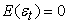;

•  have constant variance (homoscedasticity), i.e.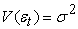;

•  are not correlated to each other (in case of the normality of the distribution are independent), i.e. the covariance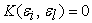for each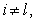.

The most important methods of regression model analysis include residual analysis. It is based on the assumption that the residuals et represent the point estimate of random errorsεt. The equationapplies, i.e. (classical) residual is the difference of empirical and theoretical values.

The assumptions, on which the model is based, are generally verified by simple graphs, respectively, using known statistical tests [4, 5].

In case of the graph displaying standardized residuals versus the theoretical values (see Figure 2), i.e. of a scatter plot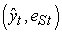applies, that the model is good if approximately 95% of residuals lies in the interval. Also residuals have to be randomly distributed around zero and the plot must not show any indication of a potential trend or pattern of development [2, 6].Download asVeiw figureFigures index
Figure 2.The dependence of standardized residuals on the theoretical values

The normality of the distribution of random errors we verified using the AndersonDarling test of goodnessoffit. On the significance level ofα = 0.05 we have tested the null hypothesisagainst the alternative hypothesis, where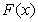is the distribution function of random selection (residuals) and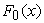is the distribution function of the normal distribution. We attained these results: AndersonDarling statistics AD = 0.5801, pvalue = 0.1217 > 0.05. Thus with a 95% reliability we can claim that random errors have a normal distribution.

Further, we tested the null hypothesis H0: random errors are uncorrelated compared to the alternative hypothesis H1: random errors are correlated. We have applied the DurbinWatson test on the significance level ofα = 0.05 with the following results: statistics DW = 2.6948, whilst the pvalue = 0.1068 > 0.05. We therefore do not reject the hypothesis on the no correlation of the random errors.

Based on these results it can be stated that model has good quality, and therefore it can be used to calculate extrapolations of the quarterly changes in the number of sold pieces of the selected articles of goods in the year 2015.

We shall use the equations (5) and (6). For the “average” of the seasonal regression parameters applies that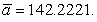For seasonal factors, we get the following results: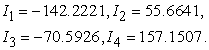(9)

The presented results can be interpreted so as the average number of sold pieces of goods annually in the first quarter decreased by about 142 pieces, in the second quarter increased by about 56 pieces, in the third quarter decreased by about 71 pieces and in the fourth quarter increased by about 157 pieces compared to the trend.

Based on the equation (4) we get for the trend estimate the relation: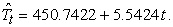(10)

Figure 3 shows a plot of the stated time series and the estimated trend.Download asVeiw figureFigures index
Figure 3. The plot of the stated time series together with the estimated trend

The extrapolated values of the original time series for each quarter of the year 2015 can be obtained based on the basis of the estimated model (8), respectively, on the basis of the respective seasonal factors (9) and the estimated trend (10).

The prediction for individual quarters of the year is as follows:or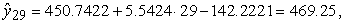oror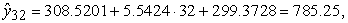or.

Now follow the application of the linear regression model (7). The good model estimated by the least squares method is:(11)

whereare unbiased estimators of the true regression coefficients β0, β1, β2, β3, β4.

Least squares parameter estimates for this model areThe predictions for individual quarters of the year 2015 are the same as in the case of application of the regression model with artificial variables: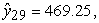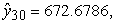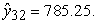The coefficient of determination is in this casetoo.Download asVeiw figureFigures index
Figure 4.The plot of the measured data with the estimated trend (11)Download asVeiw figureFigures index
Figure 5. The plot of the stated time series together with the estimated trend (11) and 95 % prediction interval

Figure 4 presents a scatter diagram of the measured data with the least squares fitted trend (11). Figure 5 shows more so the 95 % prediction interval for sold pieces of selected articles of a business company.

### 4. Conclusion

The current paper presents the analysis of time series with linear growing trend and additive seasonal component. To determine the seasonal component, a method based on the theory of linear regression model with artificial variables, i.e., variables that are discrete or qualitative in nature, so they cannot be directly quantified, was used. For eliminating seasonal component was used regression model with trigonometric functions too.

The analysis of the seasonal component allows us to increase our knowledge about the patterns of behavior of a given effect, respectively phenomenon, and contribute to the construction of better forecasts of the considered time series.

### Acknowledgement

This work was supported by the VEGA grant scheme no. 1/1205/12 Numerical Modeling of Mechatronic Systems and the VEGA grant scheme no. 1/0393/14 Analysis of Causes of Mechanical System Failures by the Quantification of Strains and Stress Fields.

### References

  Arlt, J., Arltová, M., Rublíková, E., The Analysis of Economic Time Series with Examples (in Czech), VŠE Prague, 2002.In article PubMed  Montgomery, D.C., Runger, G.C., Applied Statistics and Probability for Engineers, John Wiley & Sons, 2003.In article  Ostertagová, E., Modelling Using Polynomial Regression, Procedia Engineering, 48 (2012), p. 500-506.In article View Article  Ostertagová, E., Applied Statistics (in Slovak), Elfa, Košice, 2011, 161 pp.In article  Ostertagová, E., Applied Statistics in the Computational Environment of the MATLAB software (in Slovak), TU Košice, 2015, 175 pp.In article  Ostertagová, E., Ostertag, O., Time Series Modelling, The 4th International Conference on Modelling of Mechanical and Mechatronic Systems, Technical University of Košice, Slovak Republic, Proceedings of conference,2011, p. 380-384.In article